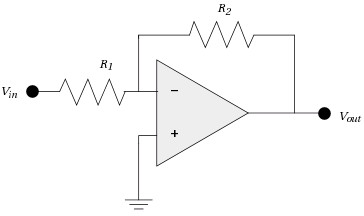## Friday, 19 December 2014

### Variations in weight change for a given Calorie change - An Engineer's Perspective.

Another techie post, inspired by Insulin Doesn't Regulate Fat Mass. Consider the inverting amplifier using an Op-Amp, below:-From HERE

As the amplifier is inverting (i.e. a ↑ input on Vin results in a ↓ output on Vout), the feedback from Vout via R2 opposes Vin via R1 at the - terminal of the Op-Amp.

If R1 = R2 and Vin changes from 0V to 1V, the change in V- (the voltage on the - terminal of the Op-Amp) varies with A (the magnitude of the Op-Amp gain) as follows*:-

A_____________Change in V-(V)
_____________0
1,000,000_____~0.000001
1,000_________~0.001
100___________~0.01
10____________~0.08
8_____________0.1
5_____________~0.14
3_____________0.2
2_____________0.25
1_____________~0.33
0_____________0.5

As the body operates on biochemical principles, slopes of input/output transfer functions aren't steep at their steepest points. E.g.From http://bja.oxfordjournals.org/content/85/1/69.long

Therefore, the gains in the various parts of the Leptin "adipostat" NFB loop are not very high. Therefore, there will be a significant variation in weight change vs Calorie change, and there will be significant variations in the variation due to loop gain variations from person to person.

Insulin Resistance makes the slopes of  the above input/output transfer functions shallower, reducing the gain in the system. This increases the variation in weight change vs Calorie change. For ways to reduce Insulin Resistance, see Insulin Resistance: Solutions to problems.

*In case anyone thinks that I've made the numbers up, here's the maths:-
Current in/out of the - terminal of the Op-Amp = 0.
∴ IR1 = IR2
I set R1 = R2 to keep the maths simple. By Ohm's Law, V = I * R.
∴ VR1 = VR2
With a 0V input:-
All currents & voltages = 0.

With a 1V input:-
VR1 = 1 - V-
VR2 = V- - Vout.  As Vout is negative, - Vout is positive.
- Vout = A * V-
∴ VR2 = V- + (A * V-)
∴ 1 - V- = V- + (A * V-)
Rearranging:-
1 = (2 * V-) + (A * V-)
Dividing both sides by V-:-
(1/V-) = 2 + A
∴ V- = 1/(2 + A)